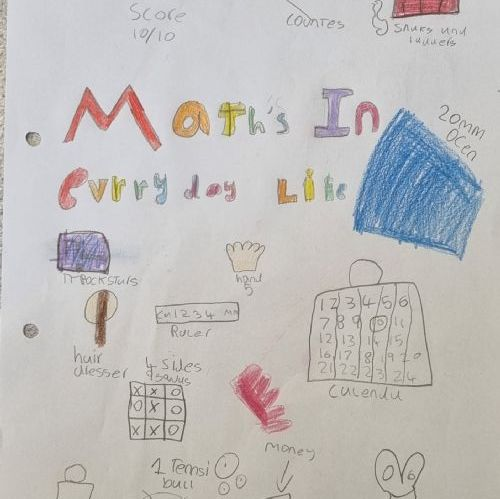# Maths and the IB Learner Profile

In our school, we foster the qualities of the IB Learner in all of our children. This is a vital aspect of maths in Kensington as, particularly with our Maths Mastery approach, the skills of the IB Learner help our children develop into strong mathematicians.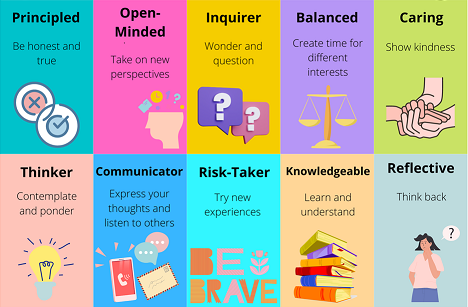We are inquirers

We ask deep, challenging questions and use maths to probe diverse topics

Our maths lessons always challenge us to make connections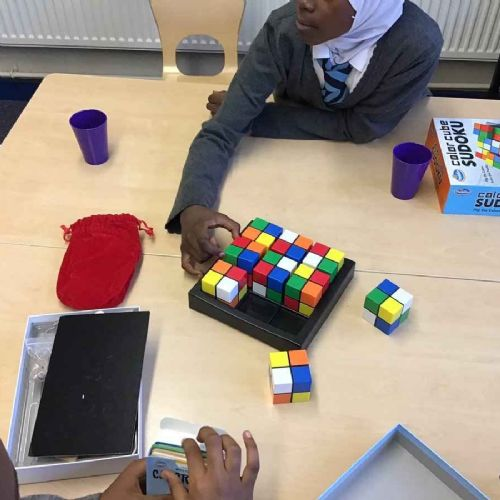We are knowledgeable

We use maths develop procedures, concepts and skills to solve familiar and unfamiliar problems

We learn key maths facts to automaticity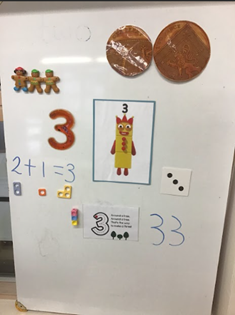We are thinkers

We evaluate information critically when reasoning and problem solving

Reasoning and problem solving are essential in our maths lessons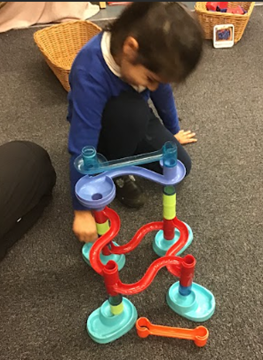We are communicators

We use accurate, technical and logical vocabulary when collaborating with others in maths

Collaboration is essential for mathematical thinking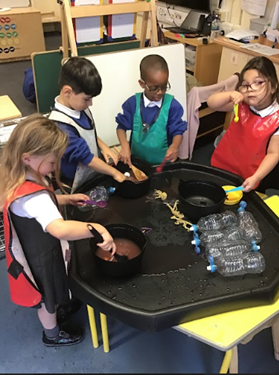We are principled

We act responsibly when using mathematical information and consistently interact with others fairly

We are open-minded

We always embrace alternative approaches to learning and using mathematics

We study a range of strategies and representations

We are caring

We respect the needs of others while offering and accepting help in maths

We use maths to support causes such as the NSPCC

We are risk-takers

We learn and use new mathematical ideas and strategies in unfamiliar contexts and take risks with our maths work

We can only be maths masters by taking risks in our work

We are balanced

We maintain other areas of interest while striving to reach our full potential in maths

We look at maths through reading and the entire curriculum

We are reflective

We consider ways to improve our learning and how mathematics affects our lives

We always look at maths in real life contexts